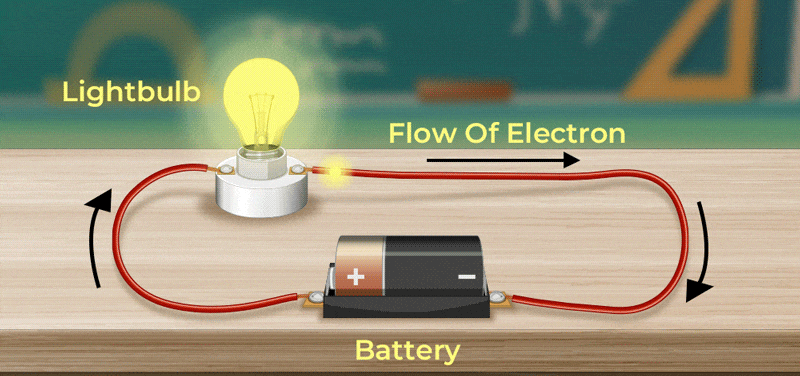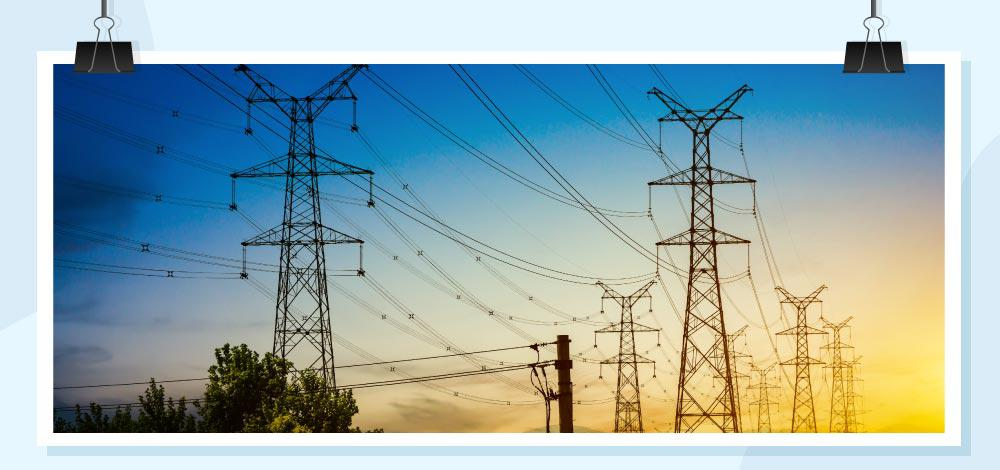GFG App
Open AppBrowser
Continue

# Electrical Energy and Power

Electric energy is the most important form of energy and is widely used in almost all the electrical devices around us. These devices have a rating written on them. That rating is expressed in Watts and intuitively explains the amount of electricity the device will consume. Bigger devices like AC, refrigerators, etc consume more electricity and thus come with a higher rating.

This rating explains the energy and power consumed by the device. It is very important to get an idea about the ratings and their meaning. Let’s learn about Electrical Energy and Electric Power in detail in this article.

## What is an Electrical Energy?

Consider a conductor with endpoints A and B, and assume that current I is flowing through the conductor. Let us denote the potential at ends by V(A) and V(B). Since the current is flowing from A to B, it means that the potential is decreasing from point A to point B.

V = V(A) – V(B)

and

V > 0

In time “t”, the charge Q travels from point A to point B.

The energy generated by the movement of the electrons from point A to B is called Electrical Energy or Electrical Potential Energy. In general, the energy derived from the kinetic and potential energy of the charged particle is called the electrical potential energy.

The image given below shows electrical energy transferred by the electron in a circuit that lits up the bulb.## Electrical Energy Formula

Let’s say the potential energy at point A is denoted by U(A) while the potential energy at point B is denoted by U(B).

U(A) = Q×V(A)

U(B) = Q×V(B)

Let the change in potential energy be denoted by Unet

Unet = Final Potential Energy – Initial Potential Energy

Unet = U(B) – U(A)

Unet = {Q×V(B) – Q×V(A)}

Unet = – ∆Q.V   (Now, I = ∆Q/∆t)

Unet = -I × ∆ t × V

If the charges inside the conductor moved freely, this potential energy would have gotten converted into kinetic energy, so that the total energy remains unchanged.

∆K = -∆U

Thus, in case the charges could move freely inside the conductor under the action of the electric field, their velocity would have increased as they move. However due to the collisions between electrons and different ions inside the conductor. The charge carriers due to not move with acceleration but with a steady velocity. During the collisions, energy is transferred from these electrons to the ions which then vibrate more vigorously, and that in turn increases the temperature. Thus the derivation is given above indirectly calculates the energy dissipated in the conductor in form of heat.

∆ W = I × V × ∆ t

## Units of Electrical Energy

Electrical energy is generally measured in joules or watt-second. When one ampere of current flows through the circuit for a second and the potential difference applied to the conductor is one volt then we say one joule of electrical energy is produced.

• SI unit for measurement of Electrical Energy is Joule. Its dimensional formula is [ML2T-2]
• Another unit for the measurement of Electrical Energy is electron-volt (eV).

### Commercial Unit of Electrical Energy

The commercial unit of measuring Electrical Energy is the kilowatt-hour (kWh) which is also known as the Board of Trade Unit (B.O.T)

• 1 kWh = 1000 × 60 × 60 watt – second
• 1 kWh = 3.6 × 106 Ws or Joules

The image given below shows how the electric power is transmitted.Note: One kWh is also called one unit

## What are Uses of Electrical Energy?

Electrical Energy has a variety of applications some of the common uses of Electrical Energy are,

• Electrical Energy is used in homes and houses to run various electrical appliances.
• Electric energy is used in cars, buses, trains, planes, and others to run music systems, AC, and other electronic systems.
• Electric cars, trains and other use electric energy for transportation.

## What are Examples of Electrical Energy?

Some examples of electrical energy are,

• Electrical energy is produced in batteries using chemical energy.
• Electric energy is produced during Lightning and Thunderstorm
• Some animals like electric eels and others generate electrical energy in their bodies and use it against predators for defense.

### Electrical Energy Into Mechanical Energy

Electrical energy can easily be converted into mechanical energy using Faraday’s law of electromagnetism.

Electric motor is the best device that converts Electrical Energy Into Mechanical Energy.

## What is Electric Power?

From the work-energy principles studied in earlier classes, it is known that power is the rate of work done. In this case, it can be thought of as the amount of energy dissipated in form of heat when an electric current is passing through the conductor. In all electrical appliances, heat dissipation occurs. This heat dissipation is called power loss or ohmic loss because these losses are due to resistances offered in conductors.

Rewriting the previous equation,

P = ∆W / t

P = I × V

Using ohm’s law relation V = IR for substituting the values inside the above equation,

P = I × V

P = I × I × R

P = I2R

It can also be written as,

P = I × V

P = (V/R) × V

P = V2/R

Thus, the power dissipated in a conductor can be written as,

• P = VI
• P = V2/R
• P = I2R

where,
P is the Power
V is the voltage applied
R is the resistance of the material
I is the current supplied

## Difference Between Electrical Energy and Electric Power

There are various differences between Electrical Energy and Electric Power some of the key differences are discussed below in the table,

## Solved Examples on Electric Energy and Power

Example 1: Find the power dissipated in a conductor with a 10V potential difference and a current of 5A.

Solution:

P = VI

Given,

V = 10
I = 5

P = VI

P = (10)(5)

P = 50 W

The power dissipated is 50 W

Example 2: Find the power dissipated in a conductor with a 5V potential difference and a current of 2A.

Solution:

P = VI

Given

V = 5
I = 2

P = VI

P = (5)(2)

P = 10 W

The power dissipated is 10 W

Example 3: An electric heater is connected to a battery of 5V potential difference. The heater has a total resistance of 50 ohms. Find the power dissipated by the electric heater.

Solution:

P = V2/R

Given,

V = 5
R = 50

P = (52)/(50)

P = 0.5 W

The power dissipated is 0.5 W

Example 4: An electric fan is connected to a battery of 20V potential difference. Assume that the fan has a total resistance of 15 ohms. Find the power dissipated by the electric fan.

P = V2/R

Given,

V = 20
R = 15

P = V2/R

P = (202)/(15)

P = 400/15

P = 26.67 W

The power dissipated is 26.67 W

Example 5: An electrical appliance is connected to a battery due to which a current of 5A flows through it. The appliance has a total resistance of 10 ohms. Find the power dissipated by the appliance.

P = I2R

Given:

I = 5
R = 10

P = (52)(10)

P = (25)(10)

P = 250 W

The power dissipated is 250 W

Example 6: An electrical appliance is connected to a battery due to which a current of 10A flows through it. The appliance has a total resistance of 20 ohms. Find the power dissipated by the appliance.

P = I2R

Given,

I = 10
R = 20

P = (102)(20)

P = (100)(20)

P = 2000 W

The power dissipated is 2000 W

## FAQs on Electric Energy and Power

### Question 1: Define Electrical Energy.

The energy passed by the electric charges in an electric field is called Electrical Energy, it can either be created by kinetic energy or potential energy of the electric charges.

### Question 2: What are the Units of Electrical Energy?

Electrical energy is measured in various units which are Joules, kilowatt-hours, electron-volt, etc.

### Question 3: What is the Commercial Unit of Electrical Energy?

The Commercial Unit of Electrical Energy is kwh or kilo-watthour

### Question 4: One unit of electrical energy equals how many joules?

One unit of electrical energy is equivalent to 3.6 × 106 joules. It is the commercial unit of electric energy.

### Question 5: What are the uses of Electrical Energy?

Electrical energy has a variety of uses some of the important uses of electric energy are,

• Electrical energy provides light energy in form of bulbs, LEDs and others.
• Electrical energy provides heat energy in form of heaters and others.
• Electrical energy provides mechanical energy in form of motors and others.

### Question 5: Define Electric Power.

The electrical energy that is transferred in an electric circuit per unit of time is called electric power, i.e. the rate of electric energy with respect to time is called Electric Power.

### Question 6: What is the SI unit of Electric Power?

The SI unit for measuring electric power is the Watt.

### Question 7: What is the formula for Electric Power?

The formula for electric power is given by,

P = VI

where,
V is the potential difference
I is the electric current
P is the electric power

### Question 8: Is Electric Power a Scalar or a Vector Quantity?

Electric power does not have any direction and hence it is a scalar quantity.

### Question 9: How is power expressed using Ohms’s law?

The formula of Power explained using Ohm’s law is

• P =I2R
• P = V2/R

where
V is the Potential Difference
I is the Electric Current
R is the Resistance
P is the Electric Power